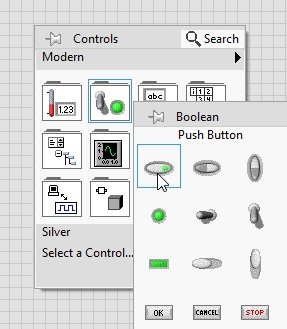# Design half adder circuit in labview : tutorial 31

### Introduction to Half adder circuit

An electronic or digital circuit that performs addition of two binary numbers and is a type of adder is known as a Half Adder. Two single binary digits are adder in a half adder and it is able to return the output plus a carry value. A simple half adder has two inputs, called A and B, and two outputs S (sum) and C (carry). The common representation uses a XOR logic gate and an AND logic gate.

### How to design half adder circuit in labview

• Lets’ design a VI, that will do simple half adder operation, in LabView. First of all create a VI as we have discussed in tutorial 1 and save it for future use as we have been doing in all the previous tutorials.
• Now we have to set to Boolean inputs for the input values A and B to be added and two Boolean outputs to display sum and carry. For Boolean inputs we will use Boolean control i.e. a push button. On the front panel click right and from the control palette select Boolean and then select Push button as shown in the figure below,Figure 1: Boolean control placement

Figure 2: Boolean indicator

Figure 3: Controls and indicators

Figure 4: Exclusive Or placement

Figure 5: Summing block

Figure 6: AND block placement

Figure 7: Carry block diagram

Figure 8: Block diagram

The output of the half adder should be according to the table given below,

 A B Sum Carry 0 0 0 0 0 1 1 0 1 0 1 0 1 1 0 1

Figure 9: Output 1

Figure 10: Output 2

Figure 11: Output 3

Figure 12: Output 4

<<Previous tutorial                                                 Next tutorial>>